nextnano.com  GUI: nextnanomat  Tool: nextnano++  Tool: nextnano³  Tool: nextnano.NEGF  Download | Search | Copyright | News | Publications  * password protected nextnano³ software1D Tight-binding band structure

# nextnano3 - Tutorial

## Empirical tight-binding (sp3s*) band structure of GaAs, GaP, AlAs, InAs, C (diamond) and Si

```  -> 1D_TightBinding_bulk_GaAs.in   -> 1D_TightBinding_bulk_GaAs_so.in   -> 1D_TightBinding_bulk_Al0.3Ga0.7As.in   -> 1D_TightBinding_bulk_GaP.in   -> 1D_TightBinding_bulk_GaP_so.in   -> 1D_TightBinding_bulk_AlAs.in   -> 1D_TightBinding_bulk_AlAs_so.in   -> 1D_TightBinding_bulk_C.in   -> 1D_TightBinding_bulk_Si.in   -> 1D_TightBinding_bulk_Ge.in   -> 1D_TightBinding_bulk_InAs_so.in   -> 1D_TightBinding_bulk_AlSb_so.in   -> 1D_TightBinding_bulk_InSb_so.in   -> 1D_TightBinding_bulk_Al0.5In0.5Sb.in```

`==> ` Download these input files (for 2015 version of nextnano³)
`==> ` Download these input files (for more recent versions of nextnano³)
`    `If you don't have a password yet, you have to first sign up for a free evaluation license in order to download these input files.

## Empirical tight-binding (sp3s*) band structure of GaAs and GaP

The empirical tight-binding model that is used here is based on the sp3s* Hamiltonian, i.e. the 10 x 10 matrix given in Table (A) of

`[Vogl]`
A semi-empirical tight-binding theory of the electronic structure of semiconductors
P. Vogl, H.P. Hjalmarson, J.D. Dow
J. Phys. Chem. Solids 44 (5), 365 (1983)

In addition, we include spin-orbit coupling leading to a 20 x 20 matrix.
The additional terms arising due to spin-orbit coupling are given for instance on p. R5 of

Microscopic theory of nanostructured semiconductor devices: beyond the envelope-function approximation
A. Di Carlo
Semiconductor Science and Technology 18, R1 (2003)

We note that nowadays much better theoretical methods are available for calculating the band structure of bulk materials.
However, for educational purposes, the chosen sp3s* method should be sufficient.

In this tutorial, we calculate the bulk band structure of

• GaAs, GaP and AlAs without    spin-orbit coupling using the parameters of` [Vogl]    `at T =    0 K
• GaAs, GaP and AlAs including spin-orbit coupling using the parameters of` [Klimeck] `at T = 300 K

`[Klimeck]`
sp3s* Tight-Binding parameters for transport simulations in compound semiconductors
G. Klimeck, R.C. Bowen, T.B. Boykin, T.A. Cwik
Superlattices and Microstructures 27 (5), 519 (2000)

## Input

The values for the tight-binding parametrization have to be specified in the input file:

``` \$numeric-control  ...  !------------------------------------------------------------------------------  ! Tight-binding parameters for GaAs (values of [Klimeck]). The units are [eV].  !------------------------------------------------------------------------------  !tight-binding-parameters = -3.53284d0             ! Esa (GaAs)                               0.27772d0             ! Epa                              -8.11499d0             ! Esc                               4.57341d0             ! Epc                              12.33930d0             ! Es_a                               4.31241d0             ! Es_c                              -6.87653d0             ! Vss                               1.33572d0             ! Vxx                               5.07596d0             ! Vxy                               0d0                   ! Vs_s_                               2.85929d0             ! Vsa_pc                              11.09774d0             ! Vsc_pa                               6.31619d0             ! Vs_a_pc                               5.02335d0             ! Vs_c_pa                               0.32703d0  0.12000d0  ! Delta_so_a Delta_so_c  ! Note: a = anion, c = cation  !       s_ = s*```

## Output

The output of the calculated tight-binding band structure can be found in the following file:
`TightBinding/BandStructure.dat`
The first column contains the number of the grid point in the Brillouin zone.
These grid points run
- from L point to Gamma point (along Lambda)
- from Gamma point to X point (along Delta)
- from X point to the U,K points
- from U,K points to Gamma point (along Sigma).
The next columns are the eigenvalues of the tight-binding Hamiltonian in units of [eV] for each grid point in k = (kx,ky,kz) space.

The file
```TightBinding/BandStructure_without_so.dat ```contains the tight-binding band structure without spin-orbit coupling.

The file
```TightBinding/k_vectors.dat ```contains for each point the information to which k point it belongs to.
``` no.  kx           ky           kz           |k|          kx[2pi/a]    ky[2pi/a]    kz[2pi/a]    |k|[2pi/a]  1    0.314159E+01 0.314159E+01 0.314159E+01 0.544140E+01 0.500000E+00 0.500000E+00 0.500000E+00 0.866025E+00  ... ```Note: Currently the units of kx, ky and kz do not take into account the lattice constant a. This should be modfied.
The values for kx, ky and kz in units of``` [2pi/a] ```are correct, however.
Another improvement would be to calculate and output the three-dimensional energy dispersion E(kx,ky,kz),
and two-dimensional slices E(kx,ky,0) through the three-dimensional energy dispersion E(kx,ky,kz) for a constant value of kz, e.g. kz = 0.

## Results

### GaAs without spin-orbit coupling

`-> 1D_TightBinding_bulk_GaAs.in`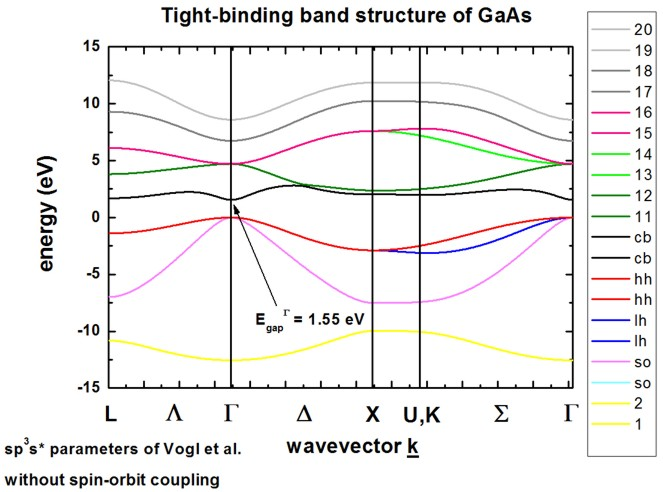The calculated band structure is in excellent agreement with Fig. 11(d) of``` [Vogl]```.
The conduction band minimum is at the Gamma point (direct band gap).
Because spin-orbit coupling is not included in the Hamiltonian,
heavy, light and split-off hole are degenerate at the Gamma point, i.e. at k = (kx,ky,kz) = 0.
The sp3s* empirical tight-binding parameters were taken from``` [Vogl] ```at T = 0 K.

### GaAs including spin-orbit coupling

`-> 1D_TightBinding_bulk_GaAs_so.in`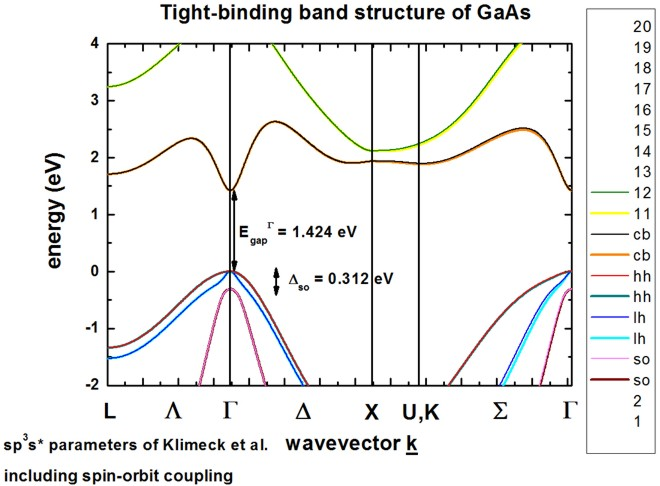The calculated band structure is in excellent agreement with Fig. 1 of``` [Klimeck]```.
The conduction band minimum is at the Gamma point (direct band gap).
Spin-orbit coupling lifts the degeneracy of heavy/light hole and split-off hole at the Gamma point.
Heavy and light hole are still degenerate at the Gamma point.
The sp3s* empirical tight-binding parameters were taken from``` [Klimeck] ```at T = 300 K.

### GaP without spin-orbit coupling

`-> 1D_TightBinding_bulk_GaP.in`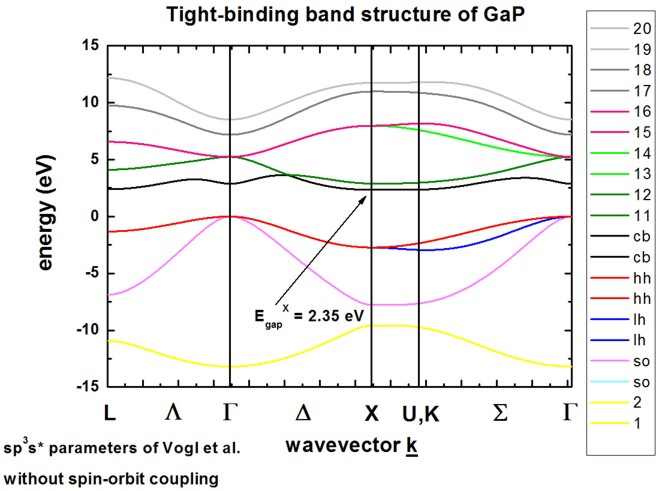The calculated band structure is in excellent agreement with Fig. 2 of``` [Vogl]```.
The conduction band minimum is calculated to be at the X point (indirect band gap).
Because spin-orbit coupling is not included in the Hamiltonian,
heavy, light and split-off hole are degenerate at the Gamma point, i.e. at k = (kx,ky,kz) = 0.
The sp3s* empirical tight-binding parameters were taken from``` [Vogl] ```at T = 0 K.

### GaP including spin-orbit coupling

`-> 1D_TightBinding_bulk_GaP_so.in`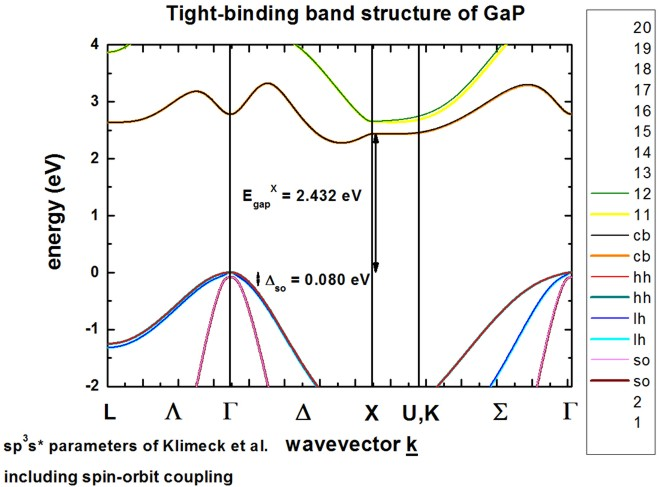The calculated band structure is in excellent agreement with Fig. 1 of``` [Klimeck]```.
The conduction band minimum is in the vicinity of the X point at the Delta line (indirect band gap), so-called camel's back.
Spin-orbit coupling lifts the degeneracy of heavy/light hole and split-off hole at the Gamma point.
Heavy and light hole are still degenerate at the Gamma point.
The sp3s* empirical tight-binding parameters were taken from``` [Klimeck] ```at T = 300 K.

### `-> 1D_TightBinding_``bulk_``AlAs.in`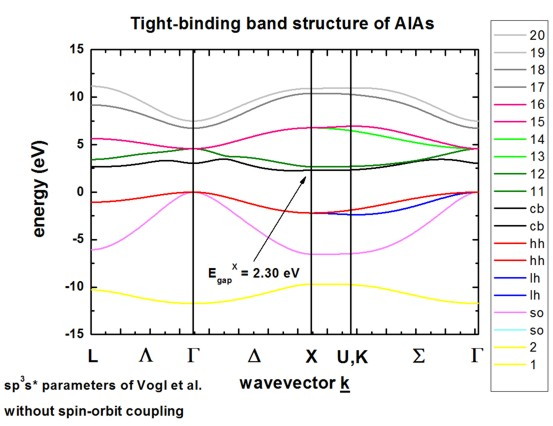### AlAs including spin-orbit coupling

`-> 1D_TightBinding_bulk_AlAs_so.in`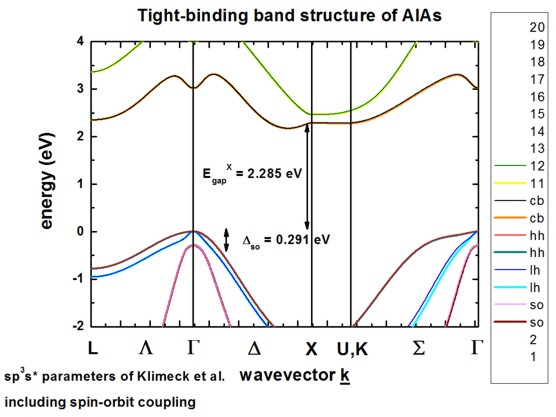### InAs including spin-orbit coupling

`-> 1D_TightBinding_bulk_InAs_so.in`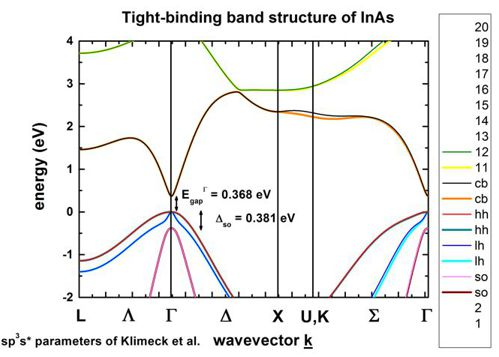### `-> 1D_TightBinding_``bulk_``C.in`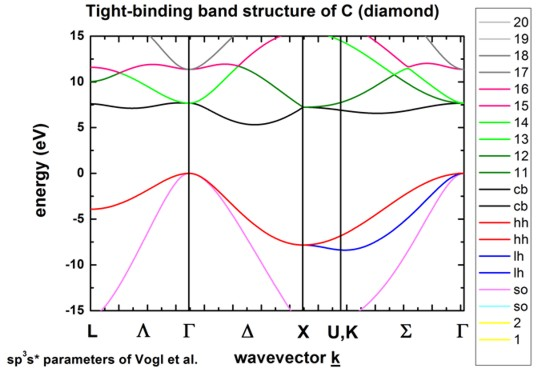### `-> 1D_TightBinding_``bulk_``Si.in`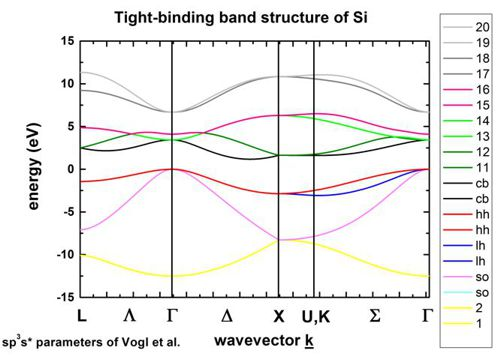The k space resolution, i.e. the number of grid points on the axis of these plots can be adjusted.

```  \$tighten    calculate-tight-binding-tighten = no              !    destination-directory           = TightBinding/    number-of-k-points              = 50              ! ```This corresponds to` 50 `k points between the Gamma point and the X point.```                                                      ! ```The number of k points along the other directions are scaled correspondingly.```  ```

• Please help us to improve our tutorial. Send comments to``` support [at] nextnano.com```.